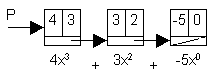Polynomials Lab, A Linked List Application

 A polynomial may be represented as a linked list where each node contains the coefficient and exponent of a term of the polynomial. For example, the polynomial 4x3 + 3x2 - 5, would be represented as shown at right.Write a program that reads two polynomials from the keyboard, stores them as linked lists, adds them together to form a third linked list, and then prints the result as a polynomial. For the polynomial above, input would be: 4 3 3 2 -5 0.

Hint: Traverse both polynomials and if a particular exponent value is present in only one of the two polynomials being summed, then it should be present in the answer. If it is present in both polynomials, then its coefficient is the sum of the corresponding coefficients in both polynomials. If this sum is zero, the term of course, should be deleted. Also, coefficient 1 and -1 is not shown unless the exponent is zero.

Use the term definition shown here on this lab.

```	struct term
{
int coef;
int exp;
term * next;
term (int C, int E, term * T)
: coef (C),
exp (E),
next T)
{  }
};
```
We have not yet indicated the order of storing the terms of the polynomial. If we allow them to be stored in any order, then it might be difficult to recognize that x5 + x2 - 3 and -3 + x5 + x2 and x2 -3 + x5 all represent the same polynomial. Hence we adopt the convention that the terms of every polynomial be stored in the order of decreasing exponents within the linked list, and we further assume that no two terms have the same exponent, and that no term has a zero coefficient.

Below are three input/output examples:

 Sample Input Expected Output

```line 1:   2   3  -4   2  -5   0
line 2:	  2   2   7   1   6   0			2x^3-2x^2+7x+1

line 1:	  4   3	 -2   1	  7   0
line 2:  -4   3   5   1  -7   0			3x

line 1:  -3   5   2   4  -1   3   1   1
line 2:   2   3  -1   1   8   0			-3x^5+2x^4+x^3+8
```
Challenge Allow inputting polynomials in any order of exponents but display the sum polynomial in descending order as above.

Continue to:  Unit 6  / Prev  / Next